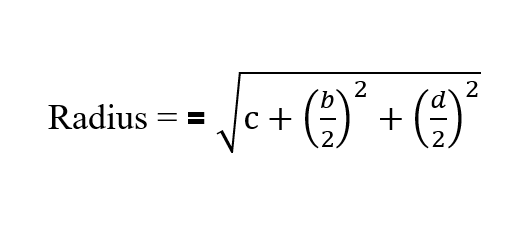Coordinate Geometry

What is coordinate geometry?

Coordinate Geometry is the study of geometry using a coordinate system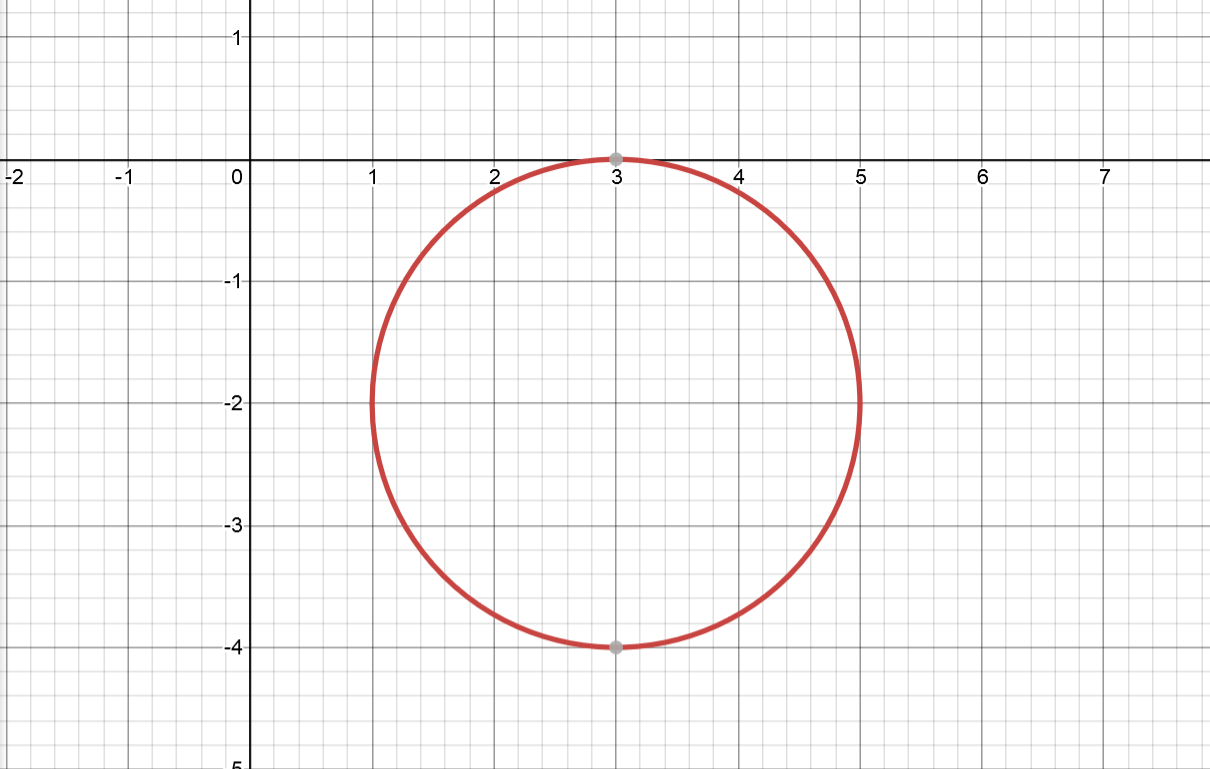What is the equation of a circle?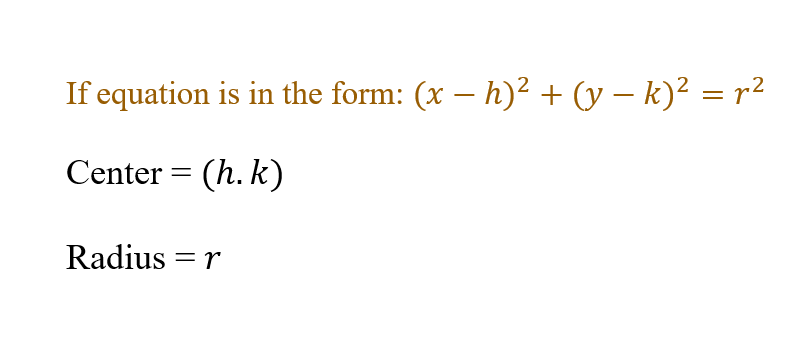Possible questions inculde: finding radius - use the equation (x-h)² + (y-k)² = r finding diameter - find radius and multiply by 2 finding the x or y coordinate of an endpoint - use the equation (x-h)² + (y-k)² = r finding the center of the circle, when the endpoints of the diameter are given - use the midpoint formula midpoint formula =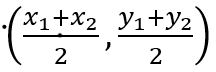Find the center of a circle whose equation is x² + bx + y² + dy = c?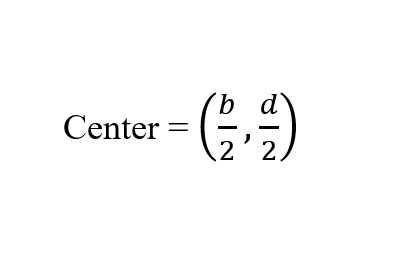Find the radius of a circle whose equation is x² + bx + y² + dy = c?